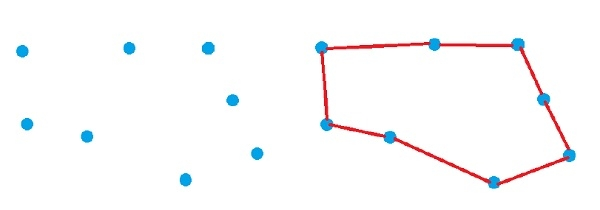# Find Simple Closed Path for a given set of points in C++

C++Server Side ProgrammingProgramming

Consider we have a set of points. We have to find a simple closed path covering all points. Suppose the points are like below, and the next image is making a closed path on those points.To get the path, we have to follow these steps −

• Find the bottom left point as P

• Sort other n – 1 point based on the polar angle counterclockwise around P, if polar angle of two points are same, then put them as the distance is shortest

• Traverse the sorted list of points, then make the path

## Example

Live Demo

#include <bits/stdc++.h>
using namespace std;
class Point {
public:
int x, y;
};
Point p0;
int euclid_dist(Point p1, Point p2) {
return (p1.x - p2.x)*(p1.x - p2.x) + (p1.y - p2.y)*(p1.y - p2.y);
}
int orientation(Point p1, Point p2, Point p3) {
int val = (p2.y - p1.y) * (p3.x - p2.x) - (p2.x - p1.x) * (p3.y - p2.y);
if (val == 0) return 0; // colinear
return (val > 0)? 1: 2; // clockwise or counterclock wise
}
int compare(const void *vp1, const void *vp2) {
Point *p1 = (Point *)vp1;
Point *p2 = (Point *)vp2;
int o = orientation(p0, *p1, *p2);
if (o == 0)
return (euclid_dist(p0, *p2) >= euclid_dist(p0, *p1))? -1 : 1;
return (o == 2)? -1: 1;
}
void findClosedPath(Point points[], int n) {
int y_min = points.y, min = 0;
for (int i = 1; i < n; i++) {
int y = points[i].y;
if ((y < y_min) || (y_min == y && points[i].x < points[min].x))
y_min = points[i].y, min = i;
}
swap(points, points[min]);
p0 = points;
qsort(&points, n-1, sizeof(Point), compare); //sort on polar angle
for (int i=0; i<n; i++)
cout << "(" << points[i].x << ", "<< points[i].y <<"), ";
}
int main() {
Point points[] = {{0, 3}, {1, 1}, {2, 2}, {4, 4},{0, 0}, {1, 2}, {3, 1}, {3, 3}};
int n = sizeof(points)/sizeof(points);
findClosedPath(points, n);
}

## Output

(0, 0), (3, 1), (1, 1), (2, 2), (3, 3), (4, 4), (1, 2), (0, 3),